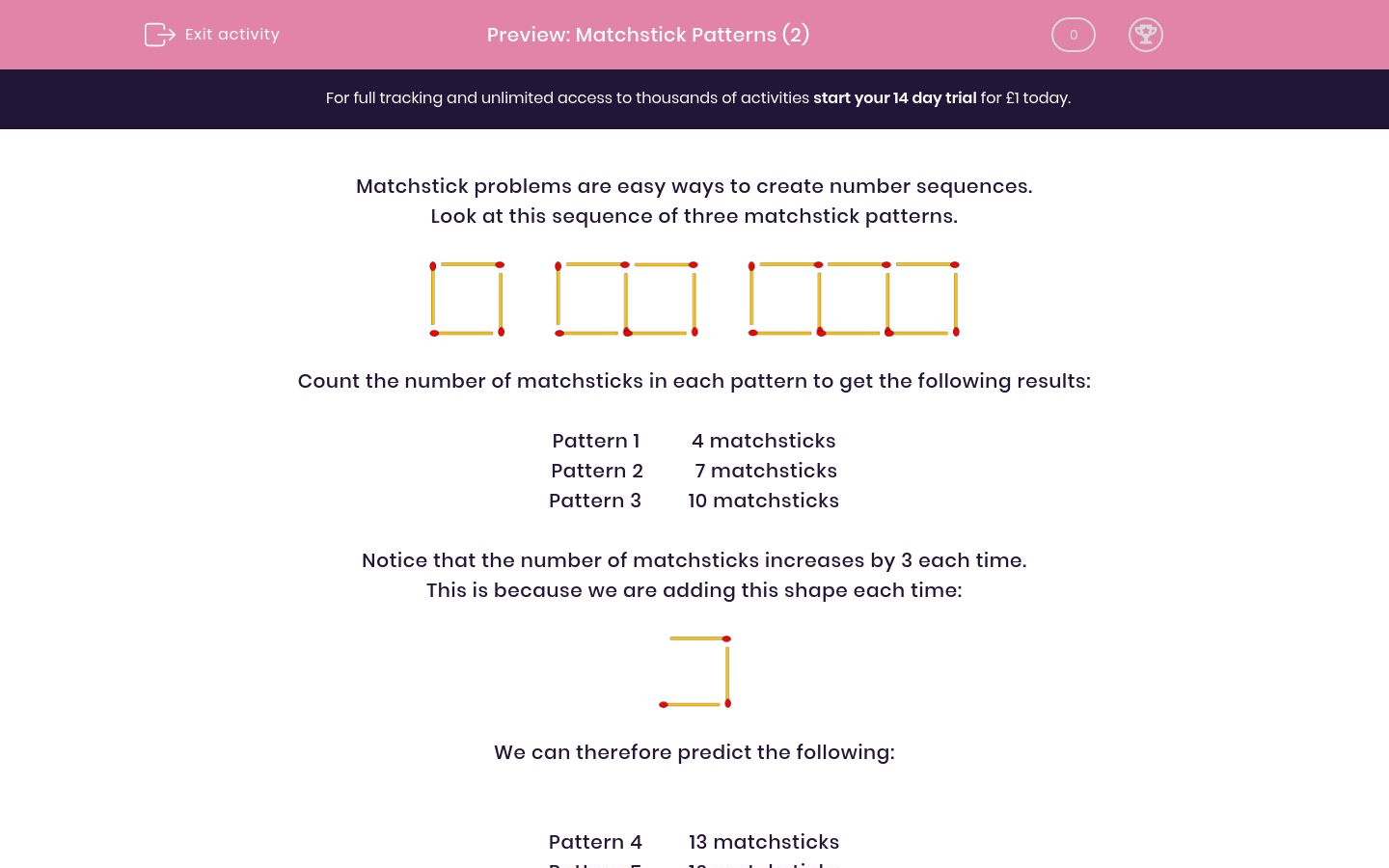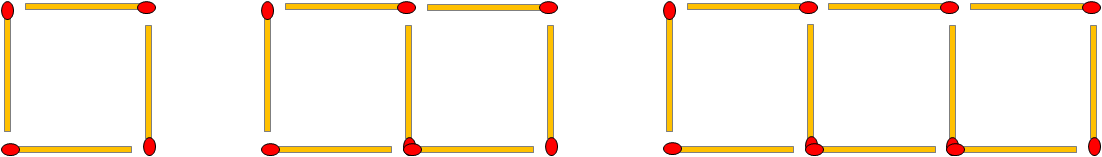# Matchstick Patterns (2)

In this worksheet, students link matchstick problems to number sequences.Key stage:  KS 2

Curriculum topic:   Maths and Numerical Reasoning

Curriculum subtopic:   Place Value

Difficulty level:### QUESTION 1 of 10

Matchstick problems are easy ways to create number sequences.

Look at this sequence of three matchstick patterns.Count the number of matchsticks in each pattern to get the following results:

Pattern 1          4 matchsticks

Pattern 2          7 matchsticks

Pattern 3         10 matchsticks

Notice that the number of matchsticks increases by 3 each time.

This is because we are adding this shape each time:We can therefore predict the following:

Pattern 4         13 matchsticks

Pattern 5         16 matchsticks

Pattern 6         19 matchsticks

Look at this matchstick pattern.How many matchsticks are there in Pattern 2 ?

Look at this matchstick pattern.How many matchsticks are there in Pattern 3 ?

Look at this matchstick pattern.How many matchsticks will there be in Pattern 4 ?

Look at this matchstick pattern.How many matchsticks will there be in Pattern 6 ?

Look at this matchstick pattern.How many matchsticks will there be in Pattern 10 ?

Look at this matchstick pattern.How many matchsticks are there in Pattern 2 ?

Look at this matchstick pattern.How many matchsticks are there in Pattern 3 ?

Look at this matchstick pattern.How many matchsticks will there be in Pattern 4 ?

Look at this matchstick pattern.How many matchsticks will there be in Pattern 5 ?

Look at this matchstick pattern.How many matchsticks will there be in Pattern 10 ?

• Question 1

Look at this matchstick pattern.How many matchsticks are there in Pattern 2 ?

15
• Question 2

Look at this matchstick pattern.How many matchsticks are there in Pattern 3 ?

22
• Question 3

Look at this matchstick pattern.How many matchsticks will there be in Pattern 4 ?

29
• Question 4

Look at this matchstick pattern.How many matchsticks will there be in Pattern 6 ?

43
• Question 5

Look at this matchstick pattern.How many matchsticks will there be in Pattern 10 ?

71
• Question 6

Look at this matchstick pattern.How many matchsticks are there in Pattern 2 ?

16
• Question 7

Look at this matchstick pattern.How many matchsticks are there in Pattern 3 ?

28
• Question 8

Look at this matchstick pattern.How many matchsticks will there be in Pattern 4 ?

40
• Question 9

Look at this matchstick pattern.How many matchsticks will there be in Pattern 5 ?

52
• Question 10

Look at this matchstick pattern.How many matchsticks will there be in Pattern 10 ?

112
---- OR ----

Sign up for a £1 trial so you can track and measure your child's progress on this activity.

### What is EdPlace?

We're your National Curriculum aligned online education content provider helping each child succeed in English, maths and science from year 1 to GCSE. With an EdPlace account you’ll be able to track and measure progress, helping each child achieve their best. We build confidence and attainment by personalising each child’s learning at a level that suits them.

Get started